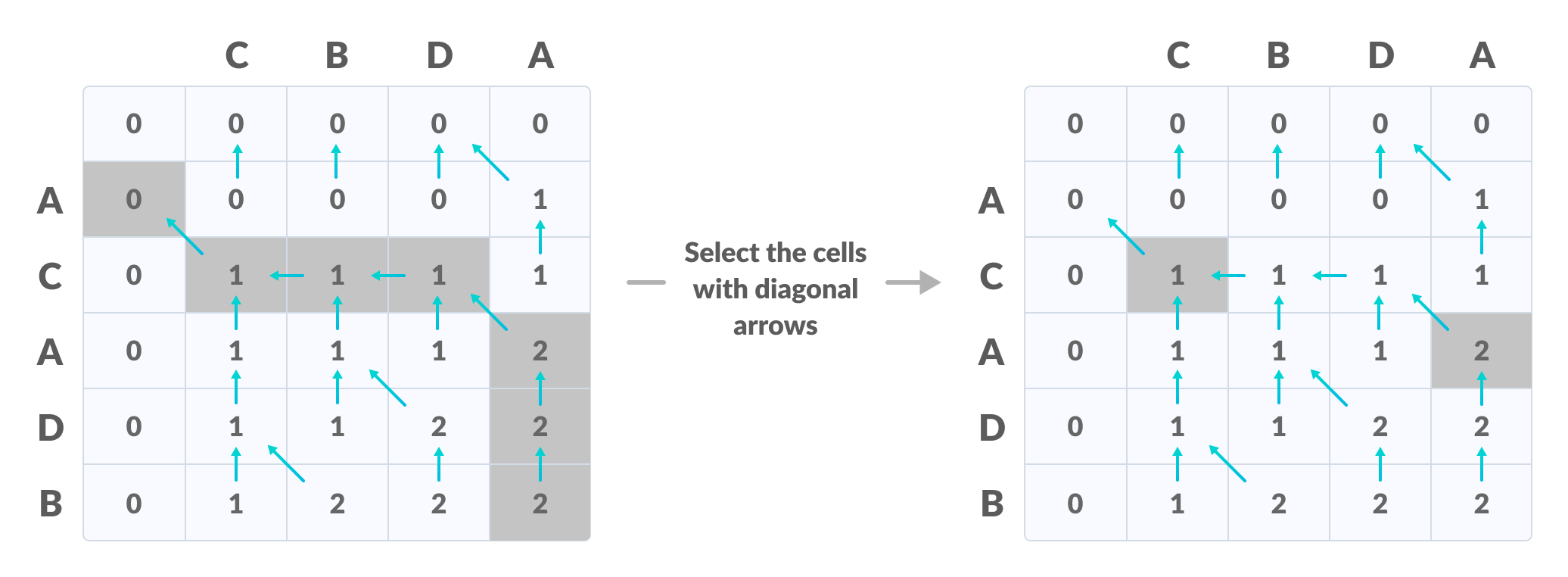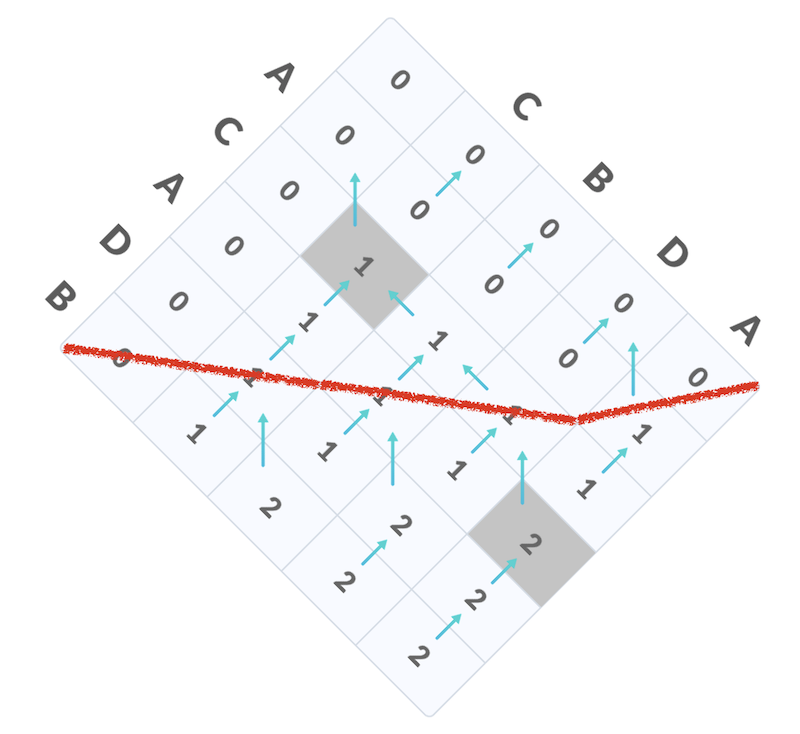# M2: 并行 Longest Common Subsequence (plcs)

#### ⏰ 截止日期

Soft Deadline: 2023 年 4 月 2 日 23:59:59。

OS2023-M2 提交结果

## 1. 背景

“最长公共子序列” (Longest Common Subsequence, LCS) 是大家熟悉的算法问题，问题的定义如下：

• $C = A' = B'$，即删除剩下的字符串相同，$C$ 被称为 $A$ 和 $B$ 的一个 “公共子序列”；
• $|C|$ 即公共子序列的长度最大。

• LCS("abcde", "ace") = 3, LCS 为 ace
• LCS("bcdaacd", "acdbac") = 4, LCS 为 cdac
• LCS("abc", "def") = 0

LCS 是一个十分经典的算法问题，也是 “动态规划” 算法的典型代表。大家可以在互联网上查找 LCS 问题的求解方法；或是在互联网上寻找讲解最长公共子序列的视频教程。

## 2. 实验描述

plcs [T]

### 2.3. 解释

• 你不能创建超过 $T$ 个线程 (main 所在的线程不计入，因此你可以利用 main 线程)

## 4. 实验指南

### 4.1. 寻找并行的机会for (int i = 0; i < N; i++) {
for (int j = 0; j < M; j++) {
// Always try to make DP code more readable
int skip_a = DP(i - 1, j);
int skip_b = DP(i, j - 1);
int take_both = DP(i - 1, j - 1) + (A[i] == B[j]);
dp[i][j] = MAX3(skip_a, skip_b, take_both);
}
}### 4.3. 实现并行求解

for (int round = 0; round < 2 * n - 1; round++) {
// 1. 计算出本轮能够计算的单元格
// 2. 将任务分配给线程执行
// 3. 等待线程执行完毕
}